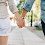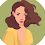# JavaScript Built-In Functions with Examples

Javascript, first introduced by Netscape, changed the static fate of the HTML web pages. Before Javascript came into existence, HTML pag...

Javascript, first introduced by Netscape, changed the static fate of the HTML web pages. Before Javascript came into existence, HTML pages were just used to render static content. Inserting Javascript to a web page, can give it a significant degree of interactivity and functionality. It lets you control the behavior of the web browser and how the elements of the web page are displayed. Most web browsers have a built in javascript interpreter. When a browser downloads an html file that contains javascript, the javascript interpreter reacts to any script.

Javascript is an object oriented programming language. It supports the concept of objects in the form of attributes. If an object attribute consists of function, then it is a method of that object, or if an object attribute consists of values, then it is a property of that object. For example,

document.write("Hello World");

write() is a method of the document object that writes the content "Hello World" on the web page. There are several Javascript built-in objects such as,
• Number
• String
• RegExp
• Array
• Math
• Date
• Boolean
Each of the above objects hold several built-in functions to perform object related functionality. Apart from these methods, Javascript provides few predefined functions which do not stick to a particular object type but are global.These global built-in functions are explained below with examples.

### isNaN()

isNaN() method determines whether value of a variable is a legal number or not.

document.write(isNan(0));
document.write(isNan("Javascript"));
document.write(isNan(-2.45));
document.write(isNan("77"));
document.write(isNan("2012/4/8"));
Results:
false
true
false
false
true

#### Note: There is a property called NaN of the Number Object which can be used to assign a variable with 'not a number' value.

var year= Number.NaN;
document.write(year);
Result:
NaN

### isFinite()

As the name indicates, this function is used to find whether a number is a finite legal number.

document.write(isFinite("5678"));
document.write(isFinite("ABCD"));
document.write(isFinite("123_456"));
Result:
true
false
false

### eval()

eval() is used to execute Javascript source code. It evaluates or executes the argument passed to it and generates output.

eval("var number=2;number=number+2;document.write(number)");
Result:
4

Note: Use of eval method is not advised for the following reasons,
* security risk
* debugging may be difficult
* may result at resource hog since each invocation of eval() creates a new instance of Javascript interpreter.

### Number()

Number() method takes an object as an argument and converts it to the corresponding number value. If the object passed cannot be converted to a number, that is if the object is not in a format to be represented as a number, then it returns NaN(not a number).

var obj1=new String("123");
var obj2=new Boolean("false");
var obj3=new Boolean("true");
var obj4=new Date();
var obj5=new String("9191 9999");

document.write(Number(obj1));
document.write(Number(obj2));
document.write(Number(obj3));
document.write(Number(obj4));
document.write(Number(obj5));
Result:
123
0
1
1342720050291
NaN

Note: For date object it returns the number of milliseconds since January 1,1970 UTC.

### String()

String() function converts the object argument passed to it to a string value.

var obj1=new Boolean(0);
var obj2=new Boolean(1);
var obj3=new Date();

document.write(String(obj1));
document.write(String(obj2));
document.write(String(obj3));
Result:
false
true
Thu Jul 19 2012 23:28:08 GMT+0530 (India Standard Time)\

### parseInt()

parseInt() function takes string as a parameter and converts it to integer.

document.write(parseInt("50"));
document.write(parseInt("77 days"));
document.write(parseInt("this is 7"));
Result:
50
77
NaN

An optional radix parameter can also be used to specify the number system to be used to parse the string argument. For example,

document.write(parseInt("10",16));
Result:
16

16 is the radix value passed, which means the string should be converted from hexadecimal to decimal value. If the radix is 8, then the string should be converted from octal to decimal value. The radix can be any number ranging from 2 to 36 that represents a numeral system.

If the radix parameter is not specified, then Javascript
• Assumes radix to be 16(hexadecimal), if the string starts with "0x"
• Assumes radix to be 8(octal), if the string starts with 0
• Assumes radix to be 10(decimal), if the string starts with any other number.

### parseFloat()

parseFloat() function takes a string as parameter and  parses it to a floating point number.

document.write(parseFloat("10.33"));
document.write(parseFloat("15 66 75"));
document.write(parseFloat("this is 77"));
document.write(pareFloat("    77    "));
Result:
10.33
15
NaN
77

Note: This function allows leading and trailing spaces. If the first character in the string is not a number, then it returns NaN. If the string has more than one set of number separated by delimiters such as spaces, semicolons,commas then it returns only the first set of number before the first delimiter.

### escape()

escape() function encodes the string passed to it so that it can be used across any network, say for example in query strings.

document.write(escape("testing escape function!!"));
Result:
testing%20escape%20function%21%21

escape() function leaves digits,latin letters and the characters + - * / . _ @ unchanged and replaces all other characters with ASCII code of the original character preceded by % symbol. Use unescape() method to decode the encoded strings.

Hope this blog helped you in identifying some useful javascript built-in functions. There are many other useful javascript functions for each built-in javascript object type. In case you want to learn about each of those functions, they are clearly explained here.

Hope this post helped you in understanding some of the useful javascript built-in functions. Please leave your comments and queries about this post in the comment sections in order for me to improve my writing skills and to showcase more useful posts.

# Subscribe to GET LATEST ARTICLES!

#### Related

JavaScript 443891146464401116

1.Good article

Any way we can get all javascript built-in functions by executing the below script.
(have a try in firebug).

for(var x in window)
{
if(typeof eval('window.' + x) == 'function')
{
console.log(eval('window.' + x));
}
}

1.Thanks for the useful info!

2.Thank You!!

3.4.wisely explained in brief..

thank you

5.6.Very well explain about java script,its very informative and knowledgble.Keep sharing such type of post...

Full stack classes in kolkata

7.Nice blog, Keep sharing some more blogs with us.

8.Nice blog and information. Keep sharing more blogs with us. Thank you.

9.10.